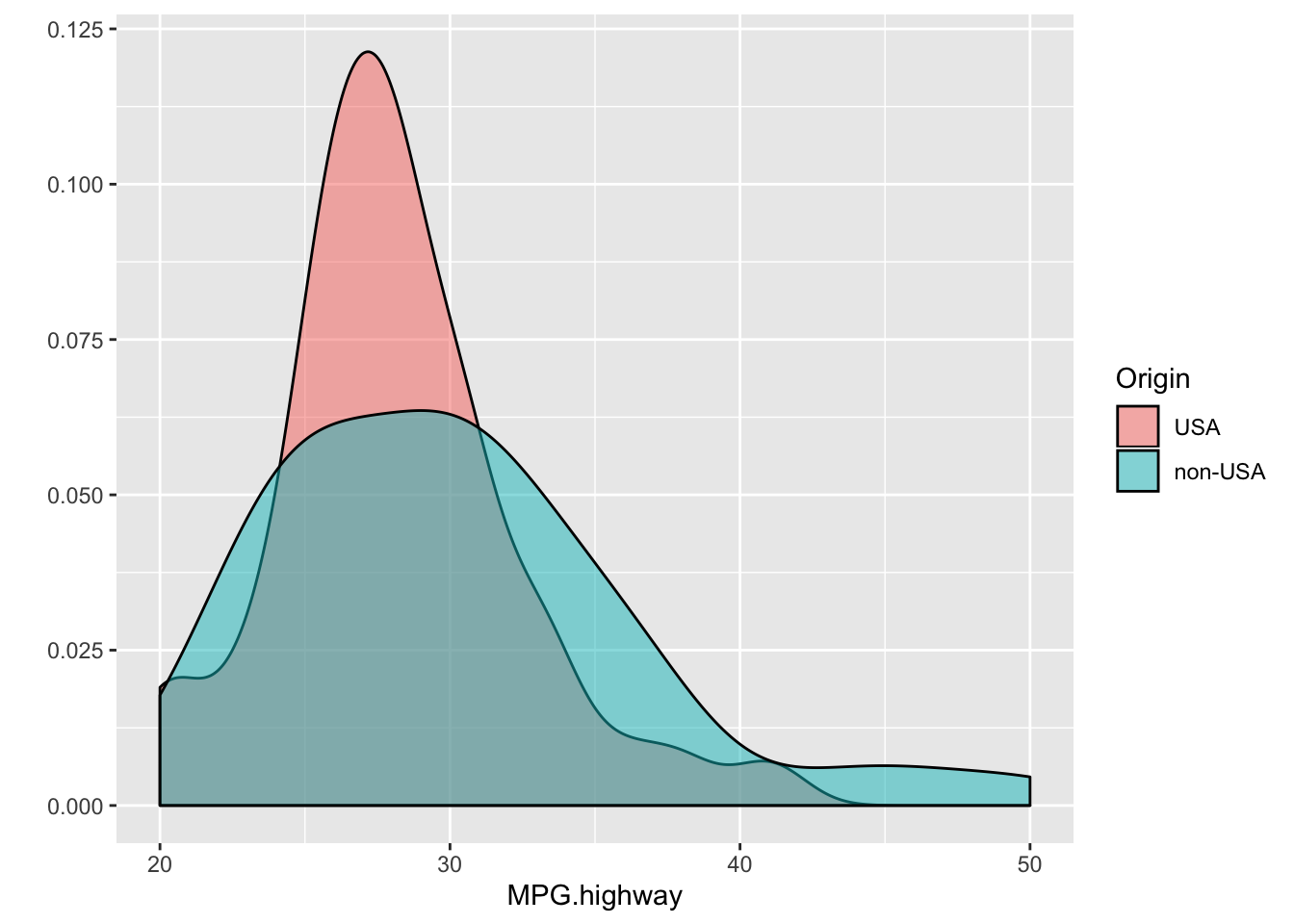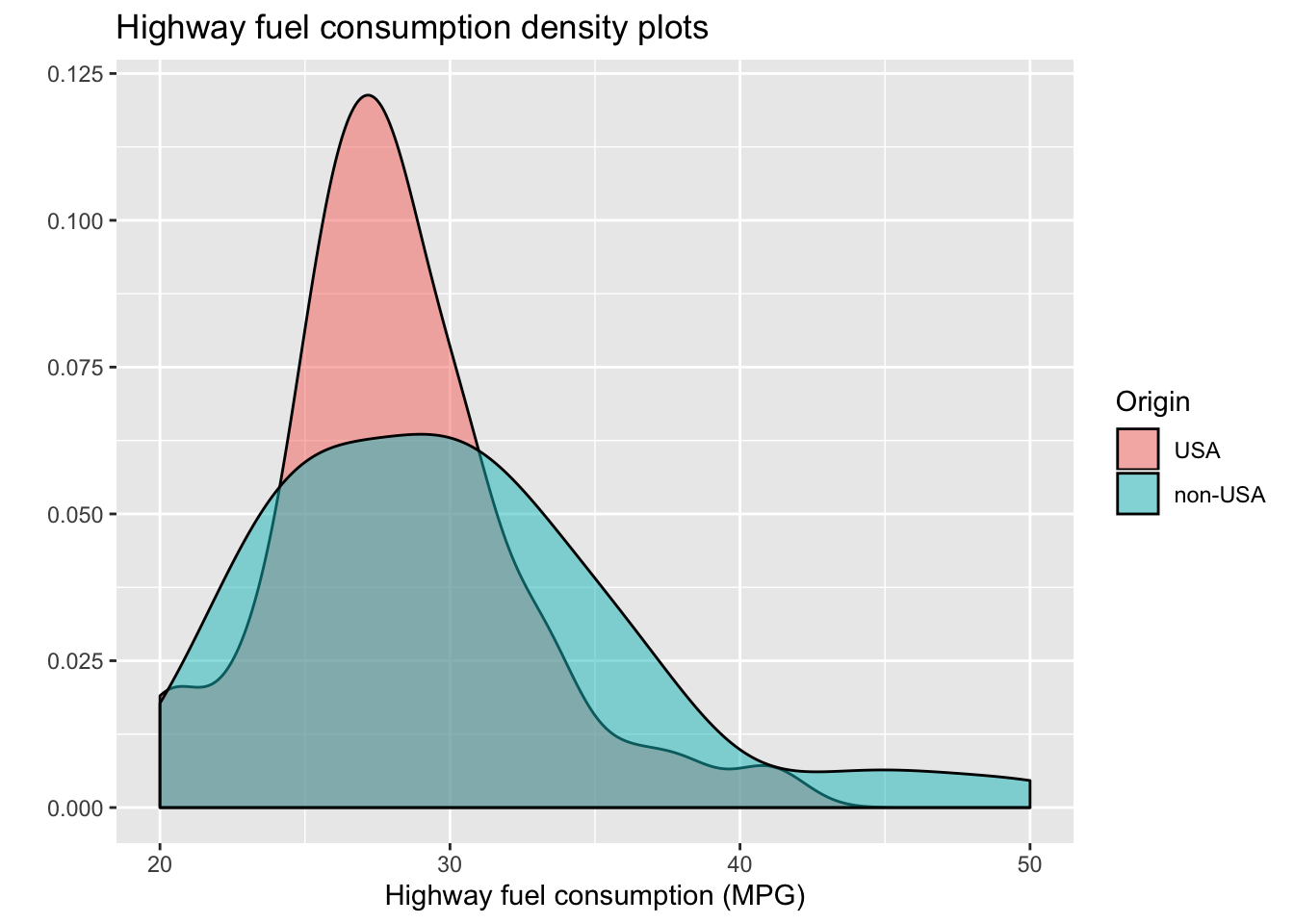##### Remember to change the `author:` field on this Rmd file to your own name.

``library(tidyverse)``
``## ── Attaching packages ──────────────────────────────── tidyverse 1.2.1 ──``
``````## ✔ ggplot2 3.2.1     ✔ purrr   0.3.3
## ✔ tibble  2.1.3     ✔ dplyr   0.8.3
## ✔ tidyr   1.0.0     ✔ stringr 1.4.0
## ✔ readr   1.3.1     ✔ forcats 0.4.0``````
``````## ── Conflicts ─────────────────────────────────── tidyverse_conflicts() ──
``Cars93 <- as_tibble(MASS::Cars93)``

### Testing means between two groups

Here is a command that generates density plots of `MPG.highway` from the Cars93 data. Separate densities are constructed for US and non-US vehicles.

``````qplot(data = Cars93, x = MPG.highway,
fill = Origin, geom = "density", alpha = I(0.5))``````(a) Using the Cars93 data and the `t.test()` function, run a t-test to see if average `MPG.highway` is different between US and non-US vehicles. Interpret the results

Try doing this both using the formula style input and the `x`, `y` style input.

``````# Formula version
mpg.t.test <- t.test(MPG.highway ~ Origin, data = Cars93)
mpg.t.test``````
``````##
##  Welch Two Sample t-test
##
## data:  MPG.highway by Origin
## t = -1.7545, df = 75.802, p-value = 0.08339
## alternative hypothesis: true difference in means is not equal to 0
## 95 percent confidence interval:
##  -4.1489029  0.2627918
## sample estimates:
##     mean in group USA mean in group non-USA
##              28.14583              30.08889``````
``````# x, y version
with(Cars93, t.test(x = MPG.highway[Origin == "USA"], y = MPG.highway[Origin == "non-USA"]))``````
``````##
##  Welch Two Sample t-test
##
## data:  MPG.highway[Origin == "USA"] and MPG.highway[Origin == "non-USA"]
## t = -1.7545, df = 75.802, p-value = 0.08339
## alternative hypothesis: true difference in means is not equal to 0
## 95 percent confidence interval:
##  -4.1489029  0.2627918
## sample estimates:
## mean of x mean of y
##  28.14583  30.08889``````

There is no statistically significant difference in highway fuel consumption between US and non-US origin vehicles.

(b) What is the confidence interval for the difference?

``mpg.t.test\$conf.int``
``````##  -4.1489029  0.2627918
## attr(,"conf.level")
##  0.95``````

(c) Repeat part (a) using the `wilcox.test()` function.

``mpg.wilcox.test <- wilcox.test(MPG.highway ~ Origin, data = Cars93)``
``````## Warning in wilcox.test.default(x = c(31L, 28L, 25L, 27L, 25L, 25L, 36L, :
## cannot compute exact p-value with ties``````
``mpg.wilcox.test``
``````##
##  Wilcoxon rank sum test with continuity correction
##
## data:  MPG.highway by Origin
## W = 910, p-value = 0.1912
## alternative hypothesis: true location shift is not equal to 0``````

(d) Are your results for (a) and (c) very different?

The p-value from the t-test is somewhat smaller than that output by wilcox.test. Since the MPG.highway distributions are right-skewed, we might expect some differences between the t-test and wilcoxon test Neither test is statistically significant.

### Is the data normal?

(a) Modify the density plot code provided in problem 1 to produce a plot with better axis labels. Also add a title.

``````qplot(data = Cars93, x = MPG.highway,
fill = Origin, geom = "density", alpha = I(0.5),
xlab = "Highway fuel consumption (MPG)",
main = "Highway fuel consumption density plots")``````(b) Does the data look to be normally distributed?

The densities don’t really look normally distributed. They appear right-skewed.

(c) Construct qqplots of `MPG.highway`, one plot for each `Origin` category. Overlay a line on each plot using with `qqline()` function.

``````par(mfrow = c(1,2))
# USA cars
with(Cars93, qqnorm(MPG.highway[Origin == "USA"]))
with(Cars93, qqline(MPG.highway, col = "blue"))
# Foreign cars
with(Cars93, qqnorm(MPG.highway[Origin == "non-USA"]))
with(Cars93, qqline(MPG.highway, col = "blue"))``````